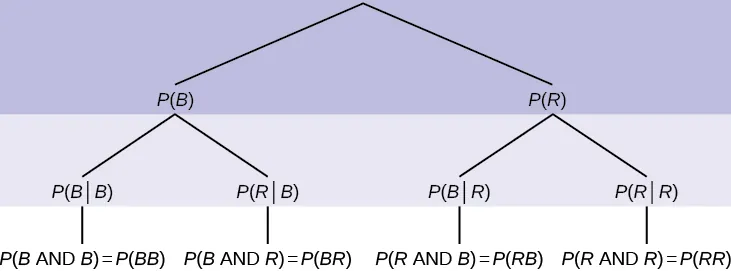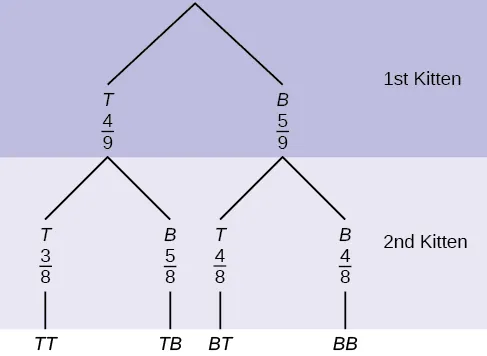Introductory Statistics

# 3.5Tree and Venn Diagrams

Introductory Statistics3.5 Tree and Venn Diagrams

Sometimes, when the probability problems are complex, it can be helpful to graph the situation. Tree diagrams and Venn diagrams are two tools that can be used to visualize and solve conditional probabilities.

### Tree Diagrams

A tree diagram is a special type of graph used to determine the outcomes of an experiment. It consists of "branches" that are labeled with either frequencies or probabilities. Tree diagrams can make some probability problems easier to visualize and solve. The following example illustrates how to use a tree diagram.

### Example 3.24

In an urn, there are 11 balls. Three balls are red (R) and eight balls are blue (B). Draw two balls, one at a time, with replacement. "With replacement" means that you put the first ball back in the urn before you select the second ball. The tree diagram using frequencies that show all the possible outcomes follows.

Figure 3.2 Total = 64 + 24 + 24 + 9 = 121

The first set of branches represents the first draw. The second set of branches represents the second draw. Each of the outcomes is distinct. In fact, we can list each red ball as R1, R2, and R3 and each blue ball as B1, B2, B3, B4, B5, B6, B7, and B8. Then the nine RR outcomes can be written as:

R1R1; R1R2; R1R3; R2R1; R2R2; R2R3; R3R1; R3R2; R3R3

The other outcomes are similar.

There are a total of 11 balls in the urn. Draw two balls, one at a time, with replacement. There are 11(11) = 121 outcomes, the size of the sample space.

#### Problem

a. List the 24 BR outcomes: B1R1, B1R2, B1R3, ...

b. Using the tree diagram, calculate P(RR).

c. Using the tree diagram, calculate P(RB OR BR).

d. Using the tree diagram, calculate P(R on 1st draw AND B on 2nd draw).

e. Using the tree diagram, calculate P(R on 2nd draw GIVEN B on 1st draw).

f. Using the tree diagram, calculate P(BB).

g. Using the tree diagram, calculate P(B on the 2nd draw given R on the first draw).

### Try It 3.24

In a standard deck, there are 52 cards. 12 cards are face cards (event F) and 40 cards are not face cards (event N). Draw two cards, one at a time, with replacement. All possible outcomes are shown in the tree diagram as frequencies. Using the tree diagram, calculate P(FF).

Figure 3.3

### Example 3.25

An urn has three red marbles and eight blue marbles in it. Draw two marbles, one at a time, this time without replacement, from the urn. "Without replacement" means that you do not put the first ball back before you select the second marble. Following is a tree diagram for this situation. The branches are labeled with probabilities instead of frequencies. The numbers at the ends of the branches are calculated by multiplying the numbers on the two corresponding branches, for example, $( 3 11 )( 2 10 )= 6 110 ( 3 11 )( 2 10 )= 6 110$.

Figure 3.4 Total = $56+24+24+6 110 = 110 110 =1 56+24+24+6 110 = 110 110 =1$

### NOTE

If you draw a red on the first draw from the three red possibilities, there are two red marbles left to draw on the second draw. You do not put back or replace the first marble after you have drawn it. You draw without replacement, so that on the second draw there are ten marbles left in the urn.

Calculate the following probabilities using the tree diagram.

#### Problem

a. P(RR) = ________

b. Fill in the blanks:

P(RB OR BR) =

c. P(R on 2nd|B on 1st) =

d. Fill in the blanks.

P(R on 1st AND B on 2nd) = P(RB) = (___)(___) = $24 110 24 110$

e. Find P(BB).

f. Find P(B on 2nd|R on 1st).

If we are using probabilities, we can label the tree in the following general way.• P(R|R) here means P(R on 2nd|R on 1st)
• P(B|R) here means P(B on 2nd|R on 1st)
• P(R|B) here means P(R on 2nd|B on 1st)
• P(B|B) here means P(B on 2nd|B on 1st)

### Try It 3.25

In a standard deck, there are 52 cards. Twelve cards are face cards (F) and 40 cards are not face cards (N). Draw two cards, one at a time, without replacement. The tree diagram is labeled with all possible probabilities.

Figure 3.5
1. Find P(FN OR NF).
2. Find P(N|F).
3. Find P(at most one face card).
Hint: "At most one face card" means zero or one face card.
4. Find P(at least one face card).
Hint: "At least one face card" means one or two face cards.

### Example 3.26

A litter of kittens available for adoption at the Humane Society has four tabby kittens and five black kittens. A family comes in and randomly selects two kittens (without replacement) for adoption.#### Problem

1. What is the probability that both kittens are tabby?

a.$( 1 2 )( 1 2 ) ( 1 2 )( 1 2 )$ b.$( 4 9 )( 4 9 ) ( 4 9 )( 4 9 )$ c.$( 4 9 )( 3 8 ) ( 4 9 )( 3 8 )$ d.$( 4 9 )( 5 9 ) ( 4 9 )( 5 9 )$
2. What is the probability that one kitten of each coloring is selected?

a.$( 4 9 )( 5 9 ) ( 4 9 )( 5 9 )$ b.$( 4 9 )( 5 8 ) ( 4 9 )( 5 8 )$ c.$( 4 9 )( 5 9 )+( 5 9 )( 4 9 ) ( 4 9 )( 5 9 )+( 5 9 )( 4 9 )$ d.$( 4 9 )( 5 8 )+( 5 9 )( 4 8 ) ( 4 9 )( 5 8 )+( 5 9 )( 4 8 )$
3. What is the probability that a tabby is chosen as the second kitten when a black kitten was chosen as the first?
4. What is the probability of choosing two kittens of the same color?

### Try It 3.26

Suppose there are four red balls and three yellow balls in a box. Two balls are drawn from the box without replacement. What is the probability that one ball of each coloring is selected?

### Venn Diagram

A Venn diagram is a picture that represents the outcomes of an experiment. It generally consists of a box that represents the sample space S together with circles or ovals. The circles or ovals represent events.

### Example 3.27

Suppose an experiment has the outcomes 1, 2, 3, ... , 12 where each outcome has an equal chance of occurring. Let event A = {1, 2, 3, 4, 5, 6} and event B = {6, 7, 8, 9}. Then A AND B = {6} and A OR B = {1, 2, 3, 4, 5, 6, 7, 8, 9}. The Venn diagram is as follows:

Figure 3.6

### Try It 3.27

Suppose an experiment has outcomes black, white, red, orange, yellow, green, blue, and purple, where each outcome has an equal chance of occurring. Let event C = {green, blue, purple} and event P = {red, yellow, blue}. Then C AND P = {blue} and C OR P = {green, blue, purple, red, yellow}. Draw a Venn diagram representing this situation.

### Example 3.28

Flip two fair coins. Let A = tails on the first coin. Let B = tails on the second coin. Then A = {TT, TH} and B = {TT, HT}. Therefore, A AND B = {TT}. A OR B = {TH, TT, HT}.

The sample space when you flip two fair coins is X = {HH, HT, TH, TT}. The outcome HH is in NEITHER A NOR B. The Venn diagram is as follows:

Figure 3.7

### Try It 3.28

Roll a fair, six-sided die. Let A = a prime number of dots is rolled. Let B = an odd number of dots is rolled. Then A = {2, 3, 5} and B = {1, 3, 5}. Therefore, A AND B = {3, 5}. A OR B = {1, 2, 3, 5}. The sample space for rolling a fair die is S = {1, 2, 3, 4, 5, 6}. Draw a Venn diagram representing this situation.

### Example 3.29

Forty percent of the students at a local college belong to a club and 50% work part time. Five percent of the students work part time and belong to a club. Draw a Venn diagram showing the relationships. Let C = student belongs to a club and PT = student works part time.

Figure 3.8

If a student is selected at random, find

• the probability that the student belongs to a club. P(C) = 0.40
• the probability that the student works part time. P(PT) = 0.50
• the probability that the student belongs to a club AND works part time. P(C AND PT) = 0.05
• the probability that the student belongs to a club given that the student works part time.
• the probability that the student belongs to a club OR works part time. P(C OR PT) = P(C) + P(PT) - P(C AND PT) = 0.40 + 0.50 - 0.05 = 0.85

### Try It 3.29

Fifty percent of the workers at a factory work a second job, 25% have a spouse who also works, 5% work a second job and have a spouse who also works. Draw a Venn diagram showing the relationships. Let W = works a second job and S = spouse also works.

### Example 3.30

#### Problem

A person with type O blood and a negative Rh factor (Rh-) can donate blood to any person with any blood type. Four percent of African Americans have type O blood and a negative RH factor, 5−10% of African Americans have the Rh- factor, and 51% have type O blood.

Figure 3.9

The “O” circle represents the African Americans with type O blood. The “Rh-“ oval represents the African Americans with the Rh- factor.

We will take the average of 5% and 10% and use 7.5% as the percent of African Americans who have the Rh- factor. Let O = African American with Type O blood and R = African American with Rh- factor.

1. P(O) = ___________
2. P(R) = ___________
3. P(O AND R) = ___________
4. P(O OR R) = ____________
5. In the Venn Diagram, describe the overlapping area using a complete sentence.
6. In the Venn Diagram, describe the area in the rectangle but outside both the circle and the oval using a complete sentence.

### Try It 3.30

In a bookstore, the probability that the customer buys a novel is 0.6, and the probability that the customer buys a non-fiction book is 0.4. Suppose that the probability that the customer buys both is 0.2.

1. Draw a Venn diagram representing the situation.
2. Find the probability that the customer buys either a novel or a non-fiction book.
3. In the Venn diagram, describe the overlapping area using a complete sentence.
4. Suppose that some customers buy only compact disks. Draw an oval in your Venn diagram representing this event.
Order a print copy

As an Amazon Associate we earn from qualifying purchases.